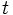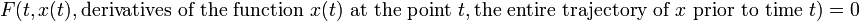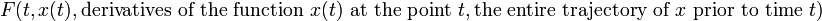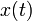# Delay differential equation

## Definition

The notion of delay differential equation (abbreviated DDE) is a variant of the notion of differential equation (in other words, delay differential equations are not (ordinary) differential equations).

### First-order first-degree case

If we denote the dependent variable by$x$ and the independent variable by$t$ (Which we think of as time), the first-order first-degree case is:$\frac{dx(t)}{dt} = f(t,x(t),\mbox{the entire trajectory of } x \mbox{ prior to time } t)$

### General case

The general case of a delay differential equation is of the form:$F(t,x(t), \mbox{derivatives of the function } x(t) \mbox{ at the point } t, \mbox{the entire trajectory of } x \mbox{ prior to time } t) = 0$

### Note on autonomous case

The delay differential equations that we study are typically autonomous delay differential equations: an equation in the general form above is autonomous if, for any$\tau \in \R$, the function$F(t,x(t), \mbox{derivatives of the function } x(t) \mbox{ at the point } t, \mbox{the entire trajectory of } x \mbox{ prior to time } t)$ is invariant under replacing$x(t)$ by the function$t \mapsto x(t - \tau)$. Intuitively, what this means is that$t$ does not appear explicitly in$F$, and all the behavior at previous points is specified in terms of how much earlier they were than$t$.